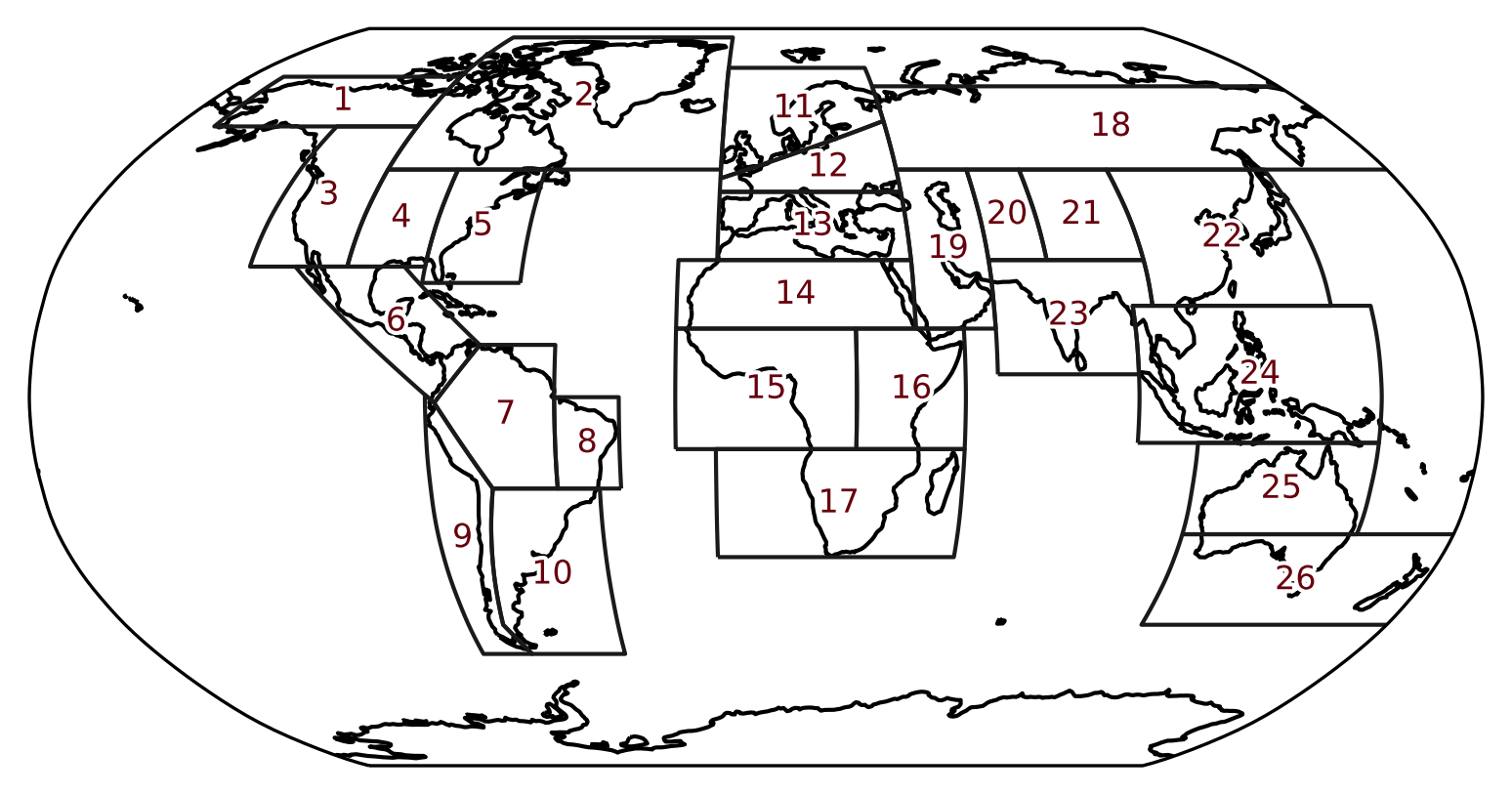None

Note

This tutorial was generated from an IPython notebook that can be accessed from github.

# Plotting

Every region has two plotting functions, which draw the outlines of all regions:

• `plot`: draws the region polygons on a cartopy GeoAxes (map)

• `plot_regions`: draws the the region polygons only

Import regionmask and check the version:

```import regionmask

```
```'0.10.0'
```

We use the srex regions to illustrate the plotting:

```srex = regionmask.defined_regions.srex
srex
```
```<regionmask.Regions 'SREX'>
Source:   Seneviratne et al., 2012 (https://www.ipcc.ch/site/assets/uploads/2...
overlap:  False

Regions:
3 WNA         W. North America
4 CNA         C. North America
5 ENA         E. North America
..  ..                      ...
22 EAS                  E. Asia
23 SAS                  S. Asia
24 SEA                S.E. Asia
25 NAU             N. Australia
26 SAU S. Australia/New Zealand

[26 regions]
```

## Plot all regions

Calling `plot()` on any region without any arguments draws the default map with a `PlateCarree()` projection and includes the coastlines:

```srex.plot();
```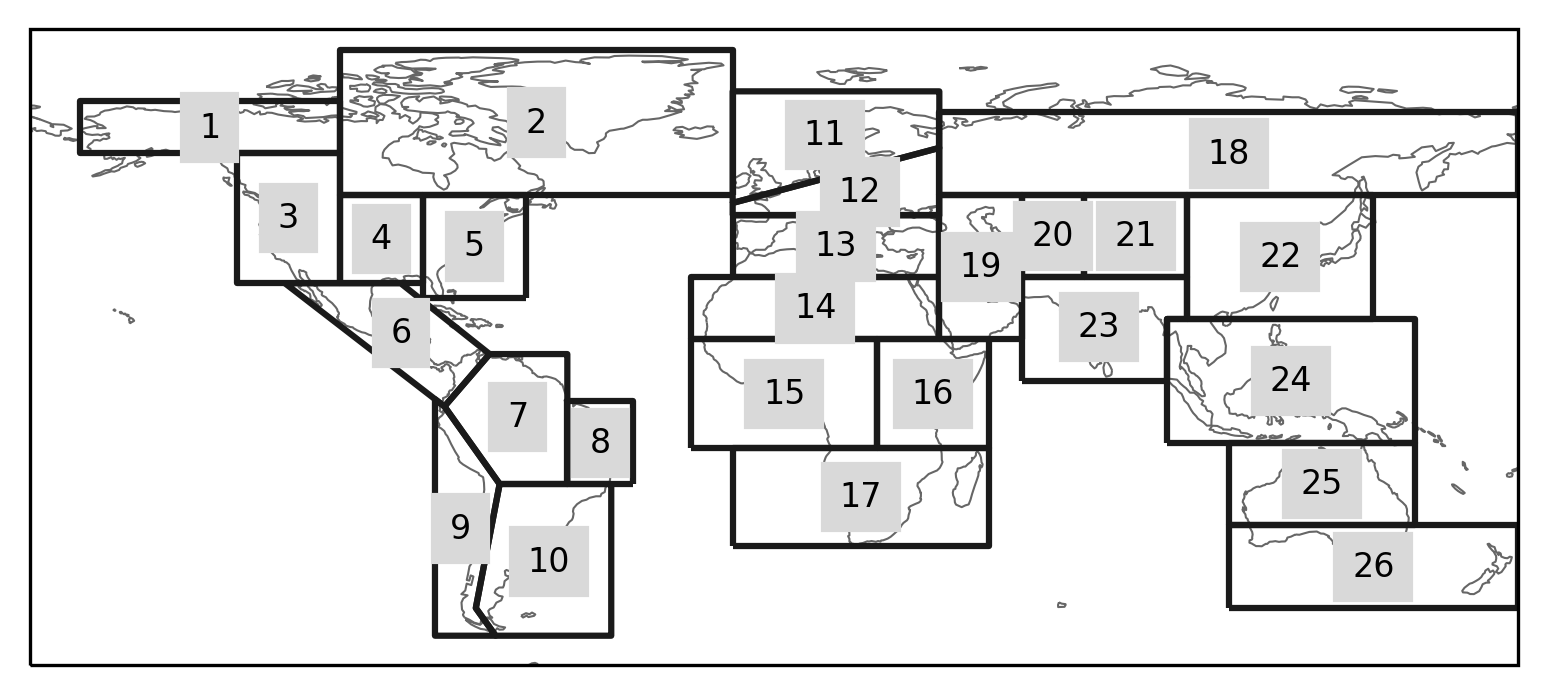## Plot options

The `plot` method has a large number of arguments to adjust the layout of the axes. For example, you can pass a custom projection, the labels can display the abbreviation insead of the region number, the ocean can be colored, etc.. This example also shows how to use `matplotlib.patheffects` to ensure the labels are easily readable without covering too much of the map (compare to the map above):

```import cartopy.crs as ccrs
import matplotlib.patheffects as pe

text_kws = dict(
bbox=dict(color="none"),
path_effects=[pe.withStroke(linewidth=2, foreground="w")],
color="#67000d",
fontsize=8,
)

ax = srex.plot(
)

ax.set_global()
```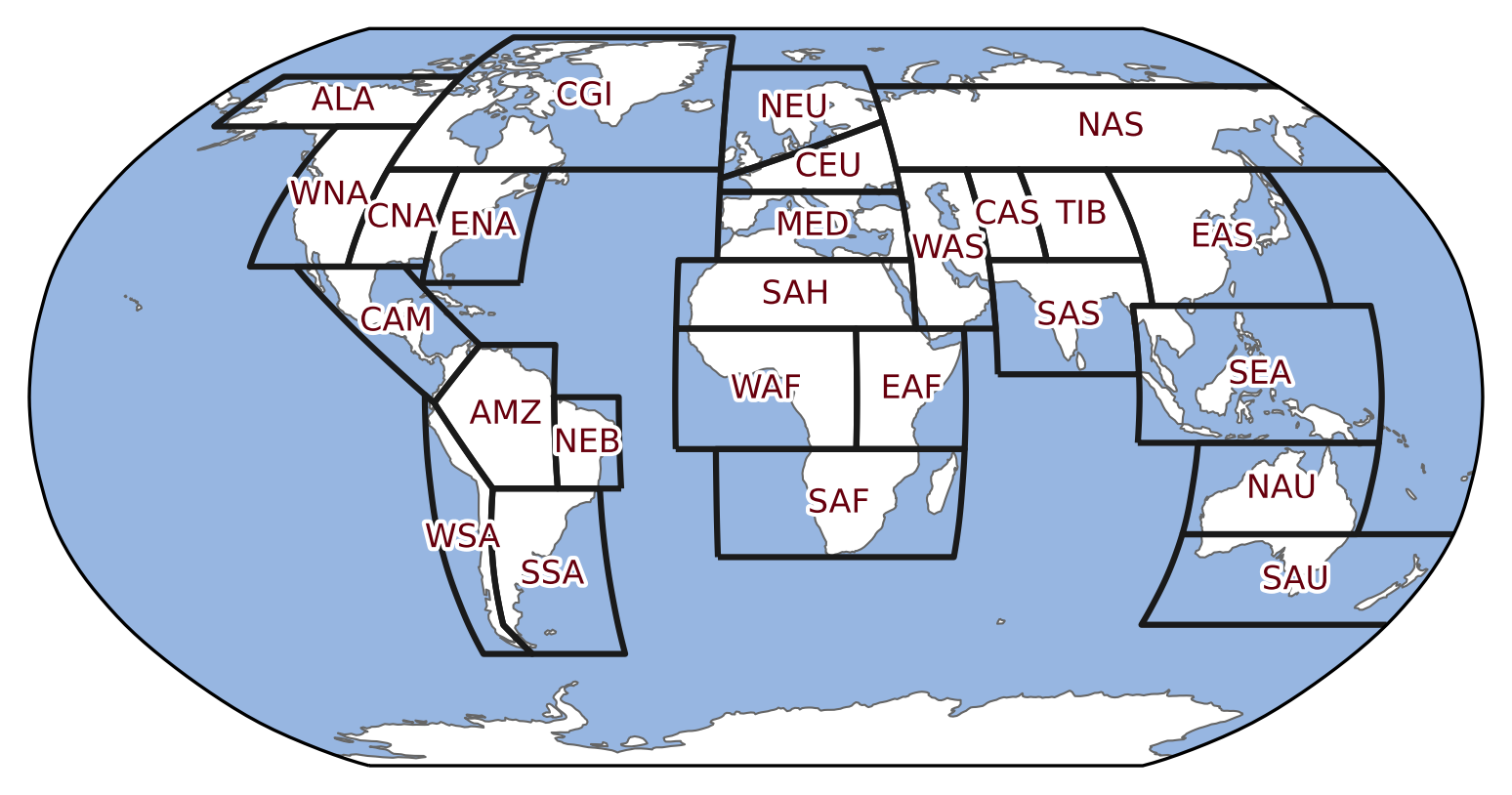## Plot only a Subset of Regions

To plot a selection of regions subset them using indexing:

```# regions can be selected by number, abbreviation or long name
regions = [11, "CEU", "S. Europe/Mediterranean"]

# choose a good projection for regional maps
proj = ccrs.LambertConformal(central_longitude=15)

ax = srex[regions].plot(
resolution="50m",
proj=proj,
label="abbrev",
text_kws=text_kws,
)

# fine tune the extent
ax.set_extent([-15, 45, 28, 76], crs=ccrs.PlateCarree())
```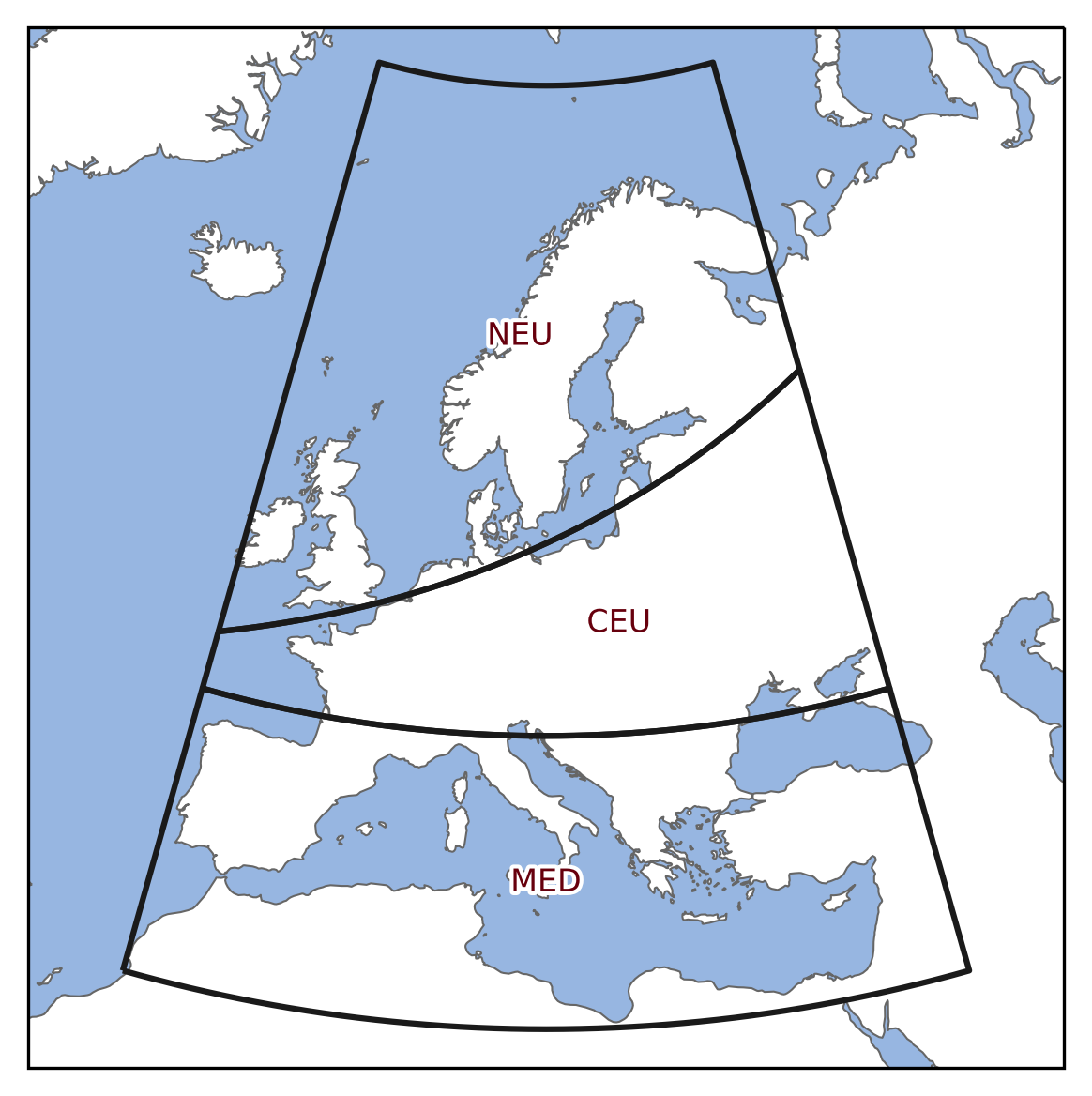## Plotting the region polygons only (no map)

```srex.plot_regions();
```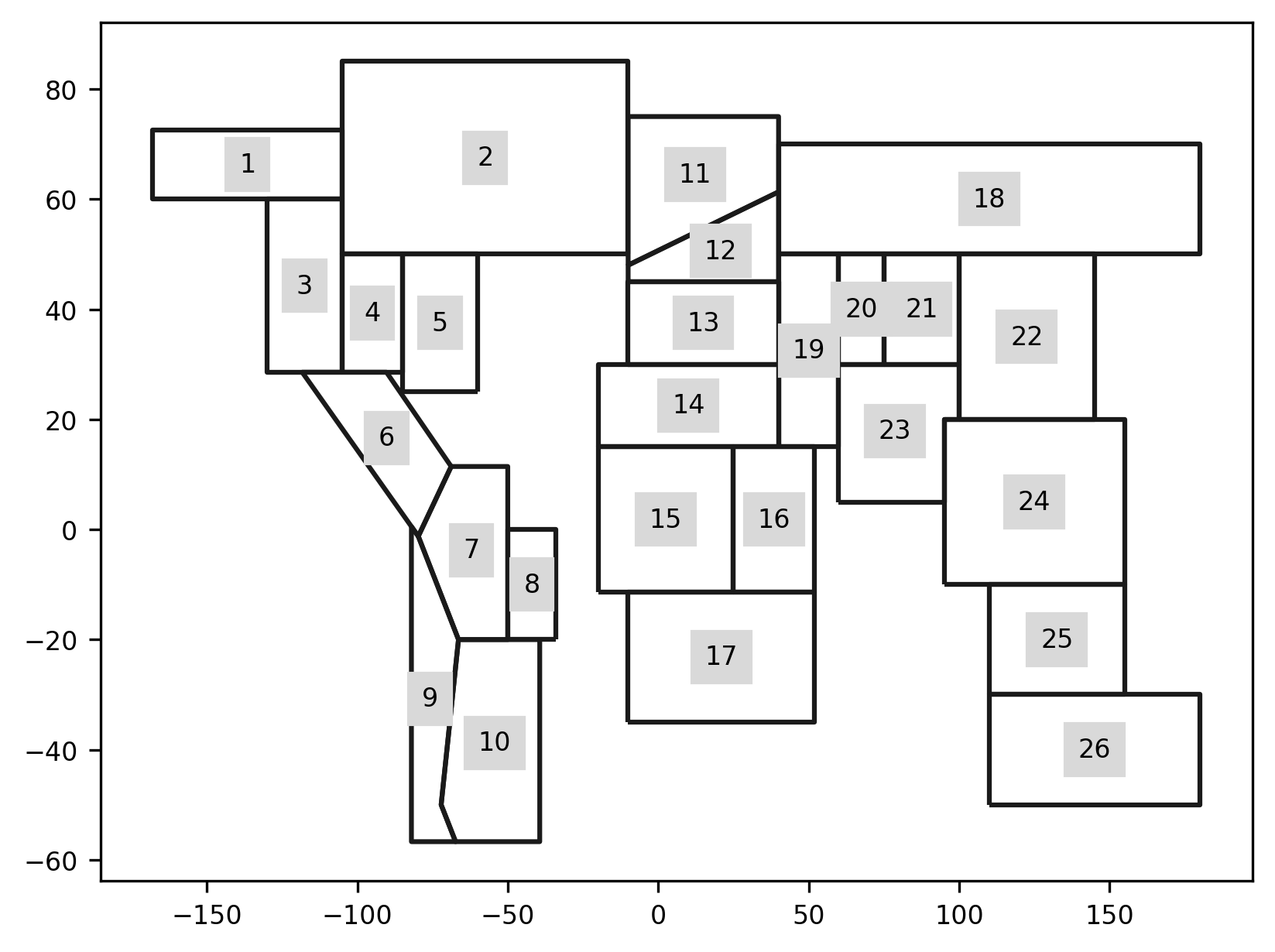Note

This does not create a cartopy GeoAxes.

To achieve this, you need to explicitly create the axes:

```import matplotlib.pyplot as plt

f, ax = plt.subplots(subplot_kw=dict(projection=ccrs.Robinson()))

srex.plot_regions(ax=ax, line_kws=dict(lw=1), text_kws=text_kws)

ax.coastlines()
ax.set_global()
```